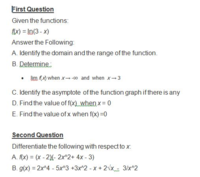# can anyone help me to answer this mathematic Question as attached.

#### ishagal1818

##### New member
First Question
Given the functions:
f(x) = ln(3 - x)
A. Identify the domain and the range of the function.
B. Determine :
• lim f(x) when x → -∞ and when x → 3
C. Identify the asymptote of the function graph if there is any
D. Find the value of f(x) when x = 0
E. Find the value of x when f(x) =0

Second Question
Differentiate the following with respect to x:
A. f(x) = (x - 2)(- 2x^2+ 4x - 3)
B. g(x) = 2x^4 - 5x^3 +3x^2 - x + 2√x - 3/x^2

#### Attachments

• 39.6 KB Views: 4

#### Subhotosh Khan

##### Super Moderator
Staff member
First Question
Given the functions:
f(x) = ln(3 - x)
A. Identify the domain and the range of the function.
B. Determine :
• lim f(x) when x → -∞ and when x → 3
C. Identify the asymptote of the function graph if there is any
D. Find the value of f(x) when x = 0
E. Find the value of x when f(x) =0

Second Question
Differentiate the following with respect to x:
A. f(x) = (x - 2)(- 2x^2+ 4x - 3)
B. g(x) = 2x^4 - 5x^3 +3x^2 - x + 2√x - 3/x^2
Please show us what you have tried and exactly where you are stuck.

Please follow the rules of posting in this forum, as enunciated at:#### ishagal1818

##### New member
I don't have idea how to solve this. I'm not good in mathematics.

#### Jomo

##### Elite Member
Do you know what domain means? Do you know what the domain of f(x) = ln (x) is? If not can you look at the graph of f(x) = ln(x) is?

Do you know how to compute the derivative of a polynomial?

#### ishagal1818

##### New member
Do you know what domain means? Do you know what the domain of f(x) = ln (x) is? If not can you look at the graph of f(x) = ln(x) is?

Do you know how to compute the derivative of a polynomial?

i dont know sir.

#### Jomo

##### Elite Member
So look it up. How can you be in this class and not know it. No one here is just going to give you the answer. You need to do some work.
The domain is the set of inputs values, usually called x-values, such that f(x) makes sense. For example if f(x) = 1/x you should know that you can't divide by so the domain is all numbers but 0.
Now look at the grapgh of y=ln x and decide what you think the domain should be.

#### Otis

##### Elite Member
… help me to answer this mathematic Question …
Hi ishagal. In a classroom, all of that material would take several days to explain. Why are you asking about these exercises? Are you reviewing past material? Are you trying to help someone else? This forum is not set up to teach classroom material. We can help you find online lecture videos, for intermediate-algebra and pre-calculus topics. Would that help?#### ishagal1818

##### New member
Im trying to get the answer for my home work.

#### Otis

##### Elite Member
Im trying to get the answer for my home work.
Are you taking an introductory calculus course? If so, then on what date did your class begin?

$$\;$$

#### tkhunny

##### Moderator
Staff member
There's the thing. Home WORK isn't same as home get-someone-else-to-do-it. You can't possibly have NOTHING to offer unless your registration in a calculus class is some sick, cruel joke being played on you by your academic advisors. Identifying Domain and Range of simple functions is the stuff of Algebra II, or so. You should not be struggling with this in Calculus. So, how did we get into this position and how can we help you without writing the whole book that you should already have mastered?

#### HallsofIvy

##### Elite Member
Oh, now, I am coming to the conclusion that many people who post questions are taking a course on "How to get other people to do your work for you"!

•Jomo